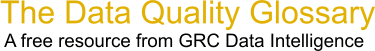Choose Index below for a list of all words and phrases defined in this glossary.

# Stakeholder Deviation

index | Index

## Stakeholder Deviation - definition(s)

Stakeholder Deviation - A statistic used to measure the variation in a distribution. Sample standard deviation is equal to the square root of (the sum of the squared deviations of the mean divided by the sample size minus 1). Where the whole population is known, the minus 1 "fudge factor" should be omitted. This "fudge factor" is degrees of freedom. Therefore, as the size of the population increases, the impact of the minus 1 "fudge factor" decreases. For a very small sample size, this minus 1 "fudge factor" can be significant.

Standard deviation can be thought of as "the average distance each data point is from the average of the entire data set". That is, add the squared distance each point is from the average value and divide by the number of points in the population, or 1 minus the number of data points for a sample, then take the square root of the answer. The distances are squared to eliminate any negative values. It is the most common measure of the variability of a set of data. If the standard deviation is based on a sampling, it is referred to as 's.' When it is describing the population, the lower case Greek letter "sigma" is used.

Although it is closely related to, and used in calculations for, the Sigma level of a process you need to be careful to distinguish the two meanings.

[Category=Data Quality ]

Source: iSixSigma, 24 February 2011 11:09:47, http://www.isixsigma.com/index.php?option=com_glossaryData Quality Glossary.  A free resource from GRC Data Intelligence. For comments, questions or feedback: dqglossary@grcdi.nl